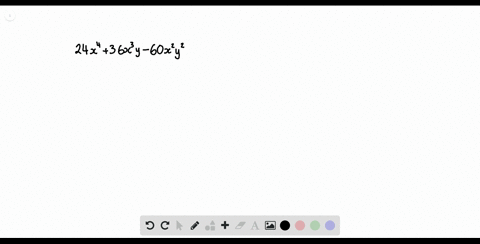Enroll in one of our FREE online STEM summer camps. Space is limited so join now!View Summer Courses### Factor each polynomial. If a polynomial cannot be…

02:46Other Schools

Need more help? Fill out this quick form to get professional live tutoring.

Get live tutoring
Problem 19

Factor each polynomial. If a polynomial cannot be factored, write prime. Factor out the greatest common factor as necessary.
$$24 a^{4}+10 a^{3} b-4 a^{2} b^{2}$$

$24 a^{4}+10 a^{3} b-4 a^{2} b^{2}=(4 a-b)(3 a+2 b)$

## Discussion

You must be signed in to discuss.

## Video Transcript

you asked a factor. This train a meal here, The first thing we d'oh is try to see if there is a common factor between all three terms. So you can see that all three terms with the fact Arata too. And we can fact you're in a square. So once that's factored out, we're left with 12 a squared plus five a b and the last term we're left with two be squared. So we're gonna write the to a squared and then we will look at this train a meal here. So from here, this is a train all meal where the leading coefficient is not one. So what we need to do is we need to multiply the leading coefficient with what would be the constant trip. So that would be p 12. And then that would be the negative, too. So if you multiply them together, we end up with negative 24. So now, identifying two factors of 24 that multiply together to be negative 24 and add together to be positive five. So that'll be a negative three positive eight. So that means we can rewrite our try no meal, and then we'll split up the five baby into positive, ate a B and negative three a B, and we'll look at it to terms at a time. So from 12 a score and the a t A B, we can't factor out for a, which means we're left with three a plus two B and now from these two terms, we can factor out a negative B and we're left with three A plus two B Once we've done that. Well, look at thes two terms and they both have the factor. Three A. Plus two b So that means we have three a plus two B as a contractor, and that means we're left with four A minus beak. And since multiplication is associative, we don't need the big rackets. We could just rewrite this as to a squared times three a plus two B times for a minus 60